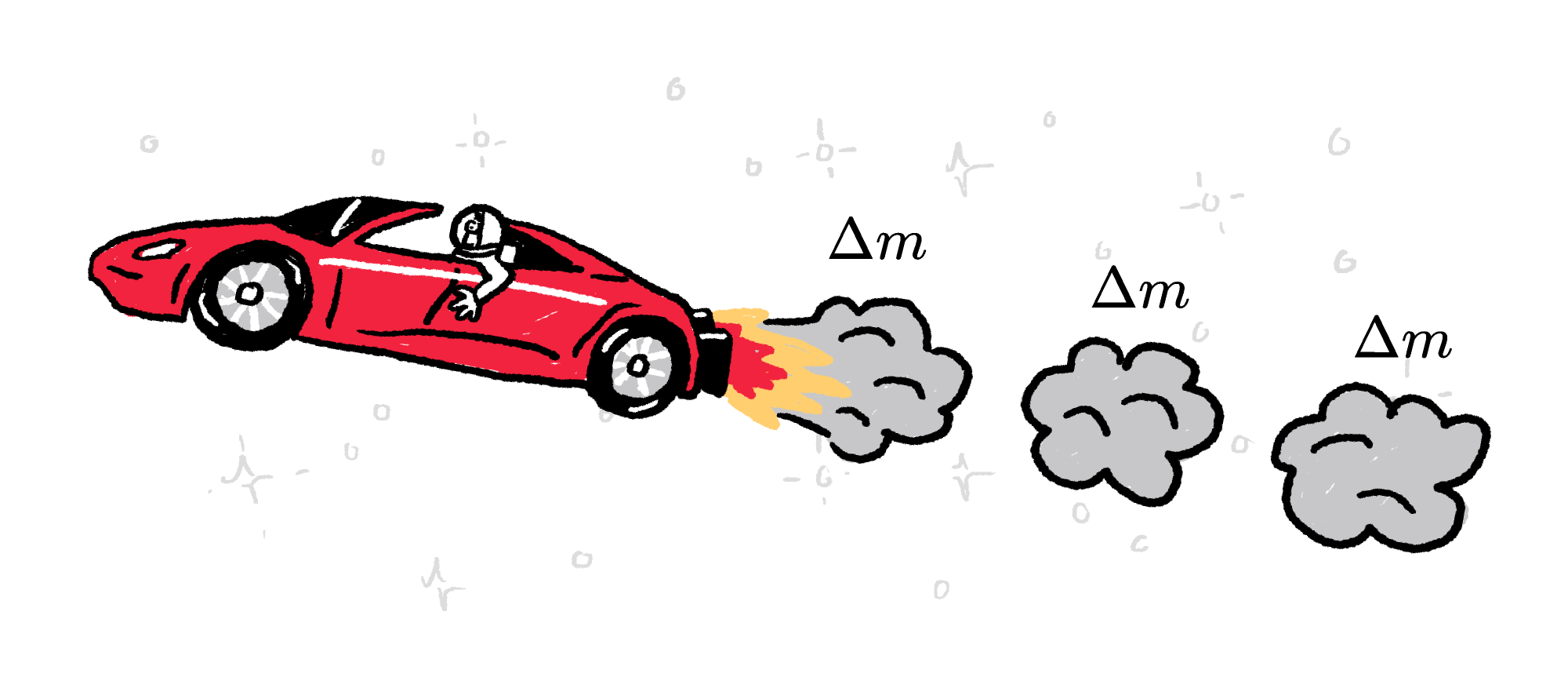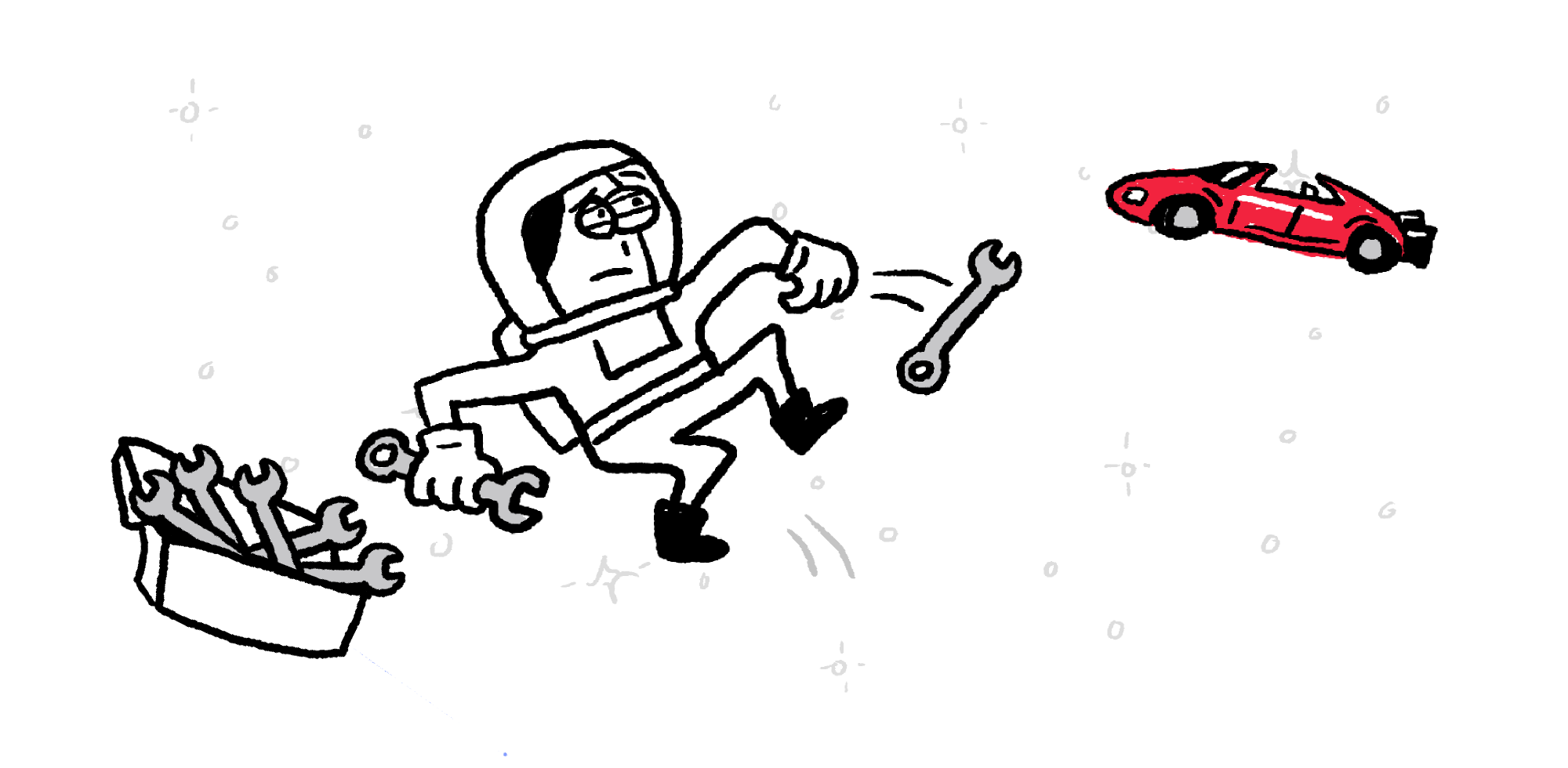Back

## Cruisin' the Solar System

To fly, birds push on the air with their wings. You push on the ground with your feet to walk. But in the vacuum of space, there's nothing to push on.

To leave Earth, a rocket pushes exhaust out of its engine as quickly as it can. By pushing exhaust downward, the rocket pushes itself upward. This thrust is also how a rocket maneuvers in space.Engineers have worked out the best fuels, the best combustion rates, and the best nozzle shapes to generate thrust efficiently. But we don’t need all that — to understand thrust, we can start by just throwing rocks.

The more massive the rock is and the faster you throw it, the more force you need to exert. But more force on the rock means more force from the rock on you, causing a greater acceleration in the opposite direction. That’s all thrust is — Newton’s third law in action.

You can explore these relationships by adjusting the sliders in the animation below.

Let’s give that idea some teeth by working out the thrust you generate when you throw a single rock with mass $m_\text{rock}$.

The change in speed before it leaves your hand depends on the force $F$ you apply and how long your throw lasts $\Delta t.$ If this force is roughly constant during the throw, it follows from Newton's law of motion and acceleration $a=\Delta v_\text{rock}/\Delta t$ that $m_\text{rock}\Delta v_\text{rock}=F\Delta t.$ This result doesn't say anything about you, only the rock. However, the rock pushes back on you with an equal force in the opposite direction. Thus, the force $F$ is exactly the thrust you generate as you launch the rock.

More specifically, $F \Delta t$ is the same for you and the rock. If your mass is $M$ and the thrust changes your speed by an amount of $\Delta v_\text{astronaut},$ then $M\Delta v_\text{astronaut}=F\Delta t.$

Since they're the same, we can replace $F\Delta t$ with $m_\text{rock}\Delta v_\text{rock}$ and find that $M\Delta v_\text{astronaut}=m_\text{rock}\Delta v_\text{rock}.$ The product of mass and the magnitude of the change in velocity, $m\Delta v,$ is the same for you and the rock.

Using a rocket-powered vehicle in space is described by exactly this relationship applied repeatedly. If the rocket expels mass $\Delta m$ each second at a speed of $v_\text{exhaust},$ you can work out how the speed of the vehicle is changing each second.In other words, you can find its acceleration. However, there's one caveat: the fuel you haven't burned yet is part of the mass of your rocket. So, as you use fuel, the mass of the rocket changes and your acceleration changes, too, even if you're burning the fuel at a constant rate.

# Today's Challenge

Our astronaut is floating $\SI{100}{\meter}$ away from her space vehicle and needs to generate some thrust to get back. She has three identical, hefty wrenches on her utility belt, so she throws one of them — but in the wrong direction!Now drifting away, she decides that the best thing to do is to come to a complete stop (with respect to the vehicle). How should she throw the second wrench?×